# Bananas plantation

Ashok Rao planted bananas on 2/7 th of his field on his 21 acers. What is the area of the bananas plantation?

B =  6 a

### Step-by-step explanation: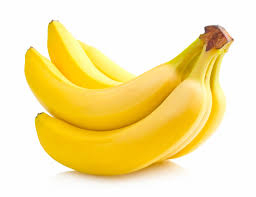Did you find an error or inaccuracy? Feel free to write us. Thank you!Tips to related online calculators
Need help to calculate sum, simplify or multiply fractions? Try our fraction calculator.

## Related math problems and questions:

• The length 5The length and breadth of rectangular field is 3 1/5 m  and 1 3/7 m. Find the area of rectangular field.
• After 2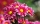After we planted flowers in 2/5 of our garden, 24m remained unplanted. How many meters is the garden in total? If the total area of the garden is 1, the proportion of the remaining area is?
• Jack planted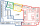Jack planted 1 1/4 rows of lettuce in the family garden, Rose planted 1 1/2 rows of broccoli and Calvin planted 4 5/12 rows of beans. How many rows were planted in the garden?
• Find the 28Find the area of a square with side length 4/5 yard.
• Fractions and mixed numerals(a) Convert the following mixed numbers to improper fractions. i. 3 5/8 ii. 7 7/6 (b) Convert the following improper fraction to mixed number. i. 13/4 ii. 78/5 (c) Simplify these fractions to their lowest terms. i. 36/42 ii. 27/45 2. evaluate following ex
• RegroupingSubtract mixed number with regrouping: 11 17/20- 6 19/20
• Field with vegetables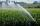Field planted with vegetables has a rectangular isosceles triangle with a leg length of 24 m. At the vertices of the triangle are positioned rotating sprinklers with a range of 12 m. How much of the field sprinkler doesn't irrigated?
• Quotient 3If the quotient of 8/13 and 2 is subtracted from the product of 1 3/4 and 8/21, what is the difference?
• DayWhat part of the day are 23 hours 22 minutes? Express as a decimal number.
• Find the 24Find the difference between 2/7 and 1/21
• Reducing scale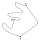I am sizing some landscaping and I measured everything for a 20 to 1 scale. I realized when I was done that it was at 30 to 1. How can I convert what I already have to 30 to 1. (Example, grass area is 22,871.6' at 20 to 1, how do you figure it out to be 3
• Fruits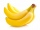For the price of one pineapple, I will buy two oranges. For the price of three oranges, I will buy four apples. I will buy 6 bananas for the price of three apples. What is the price of one banana if I pay 1 euro for one pineapple?
• Harvester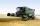Harvester has cutting width 4 m and harvested grain in the field, which is 87 meters wide. Harvester moves along the length of the field for six minutes, going back (without cutting) it takes half the time. Each turn at the end field take one minute. How
• Change the numbers in the ratio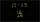Change the numbers 29, 38, and 43 in a 3: 4 ratio.
• NumbersDetermine the number of all positive integers less than 4183444 if each is divisible by 29, 7, 17. What is its sum?
• Simplest form of a fractionWhich one of the following fraction after reducing in simplest form is not equal to 3/2? a) 15/20 b) 12/8 c) 27/18 d) 6/4
• FractionsSort fractions z1 = (6)/(11); z2 = (10)/(21); z3 = (19)/(22) by its size. Result write as three serial numbers 1,2,3.